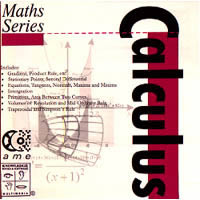# Calculus (CD-ROM)

SKU / ISBN:
Author: Dowle, Philip

• Unit price per
Tax included. Shipping calculated at checkout.

This CD explains and demonstrates the major introductory units of Calculus. A combination of worked examples, animations, video, graphics and interactive tutorials complement the text to stimulate and engage the student. The subject treatment is divided into eight parts, each with its own sub lessons:

Element Calculus - Part 1 - Introduction

1. Limits and continuity

Element Calculus - Part 2 - Gradient, Product Rule, etc.

2. Product, chain and quotient rules

Element Calculus - Part 3 - Stationary Points, Second Differential

1. Using dy/dx; stationary points
2. Calculus - second differential

Element Calculus - Part 4 - Equations, Tangents, Normals, Maxima and Minima

1. Equations, tangents and normals
2. Maxima and minima - solving problems

Element Calculus - Part 5 - Integration

1. Anti-differentiation (integration)
2. Area under a curve

Element Calculus - Part 6 - Primitives, Area Between Two Curves

1. Primitives, indefinite integrals, definite integrals
2. Area between two curves

Element Calculus - Part 7 - Volumes of Revolution and Mid-Ordinate Rule

1. Volumes of revolution
2. Mid-ordinate rule

Element Calculus - Part 8 - Trapezoidal and Simpson's Rule

1. Trapezoidal rule
2. Simpson's rule

A valuable resource for any teacher or student of Calculus, suitable for home, library or classroom use.

• Format: CD-ROM
• Publication Date: 02/01/2000
• Publisher: Knowledge Books and Software
• Subject: Maths
• Audience: Secondary